# Blog Archives

## “Increase sample size until statistical significance is reached” is not a valid adaptive trial design; but it’s fixable.

December 7, 2018
By

TLDR: Begin with N of 10, increase by 10 until p __ 0.05 or max N reached. This design has inflated type-I error. Lower p-value threshold needed to ensure specified type-I error rate. The number of interim analyses and max N affect the type-I error rate. Threshold can be identified using simulation. A recent Facebook … Continue reading "Increase...

## One-arm Bayesian Adaptive Trial Simulation Code

November 9, 2018
ByI had an opportunity recently to design a Bayesian adaptive trial with several interim analyses that allow for early stopping due to efficacy or futility. The code below implements the one-arm trial described in the great introductory article by Ben Saville et al. Three of the coauthors are current or former colleagues at Vandy. The … Continue reading One-arm...

## Quick illustration of Metropolis and Metropolis-in-Gibbs Sampling in R

June 3, 2017
By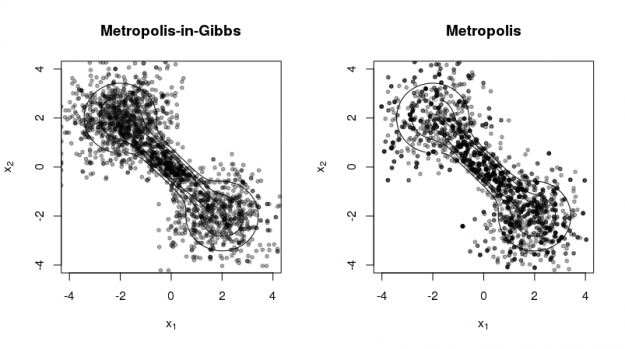The code below gives a simple implementation of the Metropolis and Metropolis-in-Gibbs sampling algorithms, which are useful for sampling probability densities for which the normalizing constant is difficult to calculate, are irregular, or have high dimension (Metropolis-in-Gibbs). ## Metropolis sampling ## x - current value of Markov chain (numeric vector) ## targ - target log … Continue reading Quick...

## Round values while preserve their rounded sum in R

July 27, 2016
By

After an embarrassing teleconference in which I presented a series of percentages that did not sum to 100 (as they should have), I found some R code on stackoverflow.com to help me to avoid this in the future. In general, the sum of rounded numbers (e.g., using the base::round function) is not the same as … Continue reading Round...

## Principal curves example (Elements of Statistical Learning)

April 21, 2016
By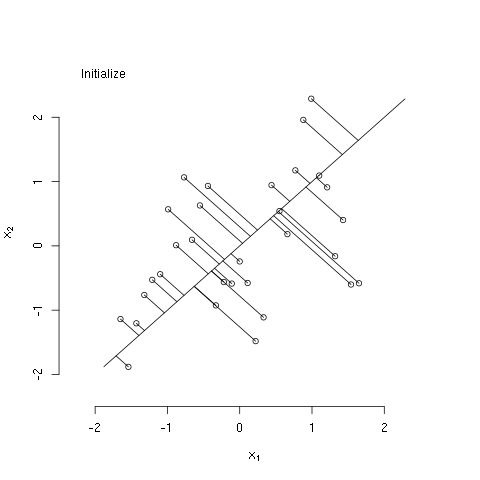The bit of R code below illustrates the principal curves methods as described in The Elements of Statistical Learning, by Hastie, Tibshirani, and Friedman (Ch. 14; the book is freely available from the authors' website). Specifically, the code generates some bivariate data that have a nonlinear association, initializes the principal curve using the first (linear) principal … Continue reading Principal...

## My Poster at Rocky 2015: Estimating parameters of the Hodgkin-Huxley cardiac cell model by integrating raw data from multiple types of voltage-clamp experiments

December 18, 2015
By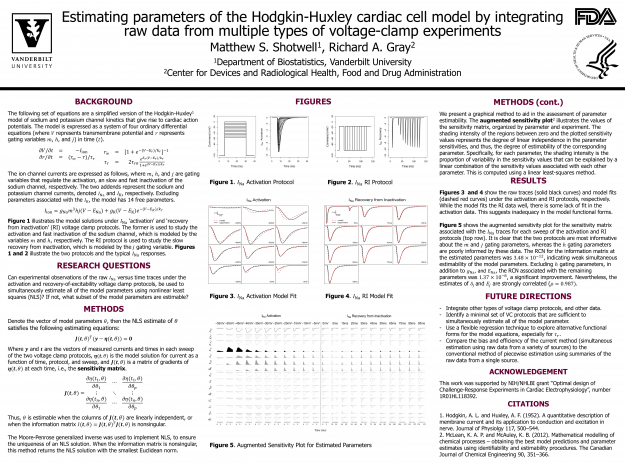I'm recently returned from the 2015 Rocky Mountain Bioinformatics Conference, where I presented the above poster. This is work with a colleague, Rick Gray, at the FDA. He and I collaborate on our NIH award "Optimal Design of Challenge-Response Experiments in Cardiac Electrophysiology" (HL118392) The (original) poster abstract is below, but the poster content is … Continue reading My...

## Reference Chart for Precision of Wilson Binomial Proportion Confidence Interval

October 16, 2015
By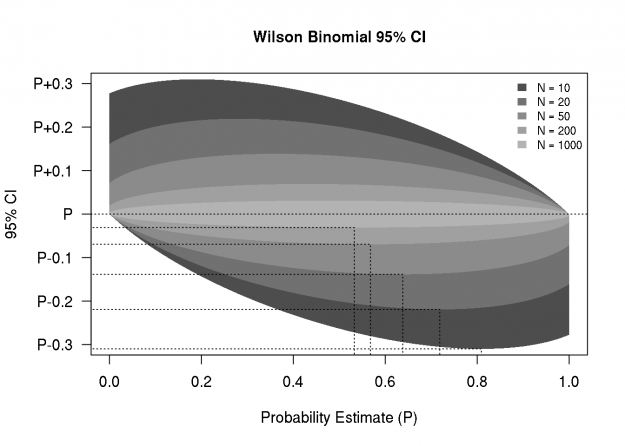I am often asked about the number of subjects needed to study a binary outcome, which usually leads to a discussion of confidence intervals for binary proportions, and the associated precision. Sometimes the precision is quantified as the width or half-width of a 95% confidence interval. For proportions, I like the Wilson score interval because … Continue reading Reference...

## Delta Method Confidence Bands for Gaussian Mixture Density (Can Behave Badly)

October 9, 2015
By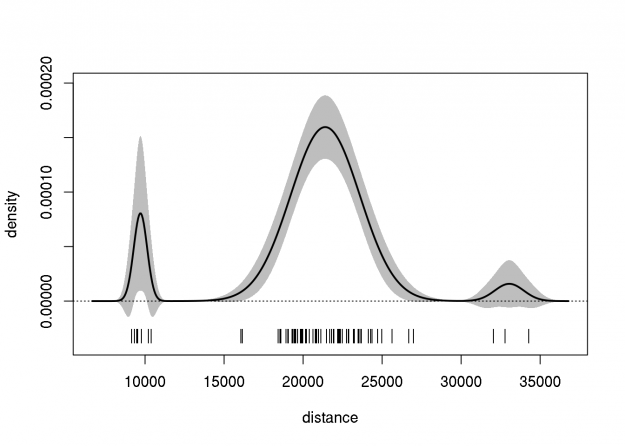This post follows from a previous post (2798), in which the delta method was used to create an approximate pointwise 95% confidence band for a Gaussian density estimate. Note that the quality of this estimate was not assessed (e.g., whether the band has the correct pointwise coverage). Here we extend that approach to the Gaussian … Continue reading Delta...

## Delta Method Confidence Bands for Gaussian Density

October 2, 2015
By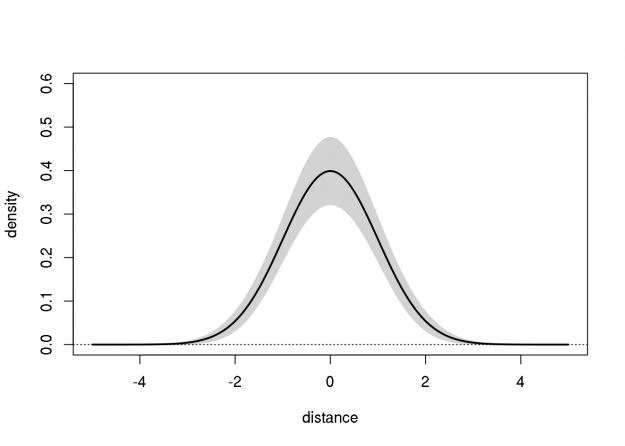During one of our Department's weekly biostatistics "clinics", a visitor was interested in creating confidence bands for a Gaussian density estimate (or a Gaussian mixture density estimate). The mean, variance, and two "nuisance" parameters, were simultaneously estimated using least-squares. Thus, the approximate sampling variance-covariance matrix (4x4) was readily available. The two nuisance parameters do not … Continue reading Delta...

## Notes on Multivariate Gaussian Quadrature (with R Code)

September 25, 2015
By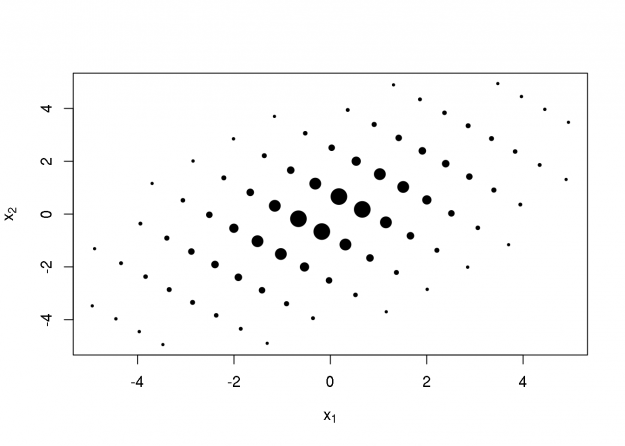Statisticians often need to integrate some function with respect to the multivariate normal (Gaussian) distribution, for example, to compute the standard error of a statistic, or the likelihood function in of a mixed effects model. In many (most?) useful cases, these integrals are intractable, and must be approximated using computational methods. Monte-Carlo integration is one